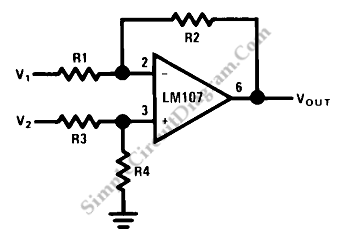# Op-Amp Circuit: Difference Amplifier

This is a circuit of difference amplifier. This circuit is an inverting amplifier that allows the subtraction of two voltages and that is the summing amplifier’s complement.This circuit also allows the the cancellation of a signal common to the two inputs.This circuit can be used as a computational amplifier, in in rejecting a common mode signal or in making a differential to single-ended conversion. Here is the circuit :The circuit bandwidth calculation is same with the banwidth calculation for inverting amplifier. This circuit has two input impedance that is not necessarily same. Non-inverting input impedance is the sum of R3 and R4 and inverting input impedance is the same as for the inverting amplifier. The gain of this circuit is the ratio of R1 to R2 for the special case of a differential input single-ended output where R1 = R3 and R2 = R4. We can use the amplifier bandwidth as basic to choose the compensation. We should be exercised in applying this circuit because input impedance are not equal for minimum bias current error. [Source: National Semiconductor Application Note]